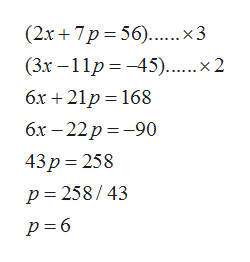# For the pair of supply-and-demand equations, where x represents the quantity demanded in units of 1000 and p is the unit price in dollars, find the equilibrium quantity and the equilibrium price.2x + 7p − 56 = 0 and 3x − 11p + 45 = 0equilibrium quantity      unitsequilibrium price    \$

Question

For the pair of supply-and-demand equations, where x represents the quantity demanded in units of 1000 and p is the unit price in dollars, find the equilibrium quantity and the equilibrium price.

2x + 7p − 56 = 0 and 3x − 11p + 45 = 0
 equilibrium quantity units equilibrium price \$
check_circleExpert Solution
Step 1

For finding the equillibrium quantity and equallibrium price, solve the both equation and find the value of p and x from equation,

Step 2

Multiplying by 3 in equation (1) and by 2 in equation (2) and on solving,help_outlineImage Transcriptionclose(2х+ 7р%3D56)....х3 (Зх — 11р %3D —45)...... x 2 бх + 21р—168 бх — 22р %3-90 43р%3D 258 р3 258/43 р -6 fullscreen
Step 3

Putting value of p in...

### Want to see the full answer?

See Solution

#### Want to see this answer and more?

Solutions are written by subject experts who are available 24/7. Questions are typically answered within 1 hour*

See Solution
*Response times may vary by subject and question
Tagged in

### Equations and In-equations